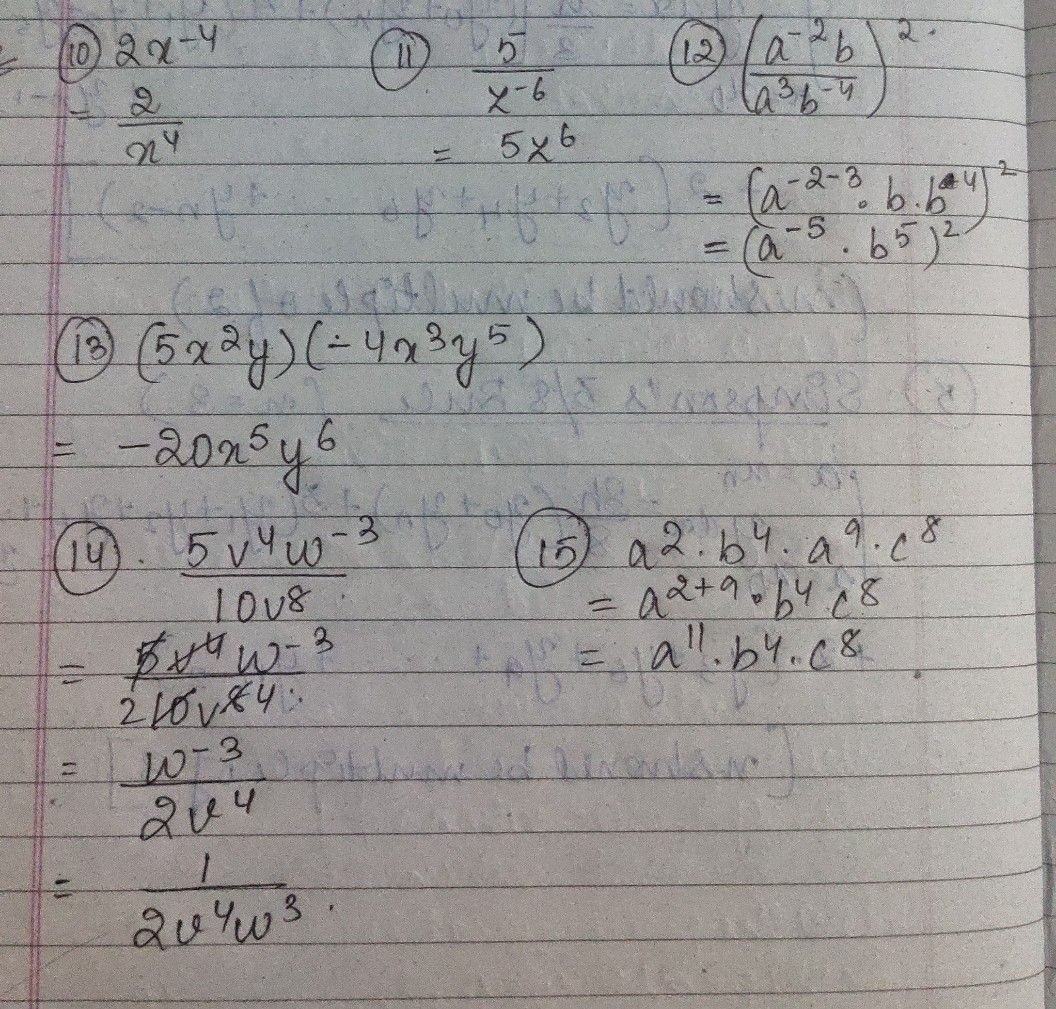Symbol
Problem$10.2x^{-4}$ $11.\dfrac {5} {z^{-6}}$ $\bar{12.\left(\dfrac {a^{-2}b} {a^{3}b^{-4}}\right)^{2}}$ $13$ $\left(5x^{2}y\right)\left(-4x^{3}y^{5}\right)$ $\bar{14.\dfrac {5v^{4}w^{-3}} {10v^{8}}}$ $15.a^{2}.b^{4}.a^{9}.c^{8}$
7th-9th grade
Algebra
SolutionQanda teacher - AnnaBell